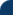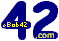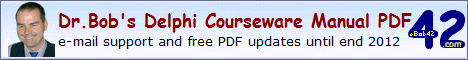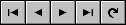Delphi Clinic C++Builder Gate Training & Consultancy Delphi Notes Weblog Dr.Bob's WebshopDr.Bob Examines... #106 See Also: Dr.Bob's Delphi Papers and ColumnsCombining Anonymous Methods and Generics
In this article, I'll examine Delphi 2009 Generics and Anonymous Methods, and especially the combination of the two. As useful example, and using some of the information from Generics.Default, let's examine two generic methods that can pick the minimum or maximum item (value) from a list of items. Where the type of the items is of course a parameter, which means that the method of determining the minimum of maximum value is also somewhat fuzzy: we need to define some way to determine which of two values of AnyType is the bigger one. This latter will be done with anonymous methods - to be passed at the time of calling (i.e. when needed).
First of all, take a look at the definition of the two generic class methods Min and Max:

```  type
TGeneric = class
class function Min<AnyType>(const values: array of AnyType;
const Comparer: IComparer<AnyType>): AnyType;
class function Max<AnyType>(const values: array of AnyType;
const Comparer: IComparer<AnyType>): AnyType;
end;
```
Note the IComparer interface, which is defined in Generics.Defaults.pas (I could also have defined my own interface, but it felt "right" to use a pre-defined approach here).
The implementation of Min and Max use this IComparer interface, which exposes a Compare method that takes two arguments (a left side and a right side) and returns a value > 0 is the left side is bigger, or a value < 0 if the left side is smaller than the right side:
```  class function TGeneric.Max<AnyType>(const values: array of AnyType;
const Comparer: IComparer<AnyType>): AnyType;
var
item: AnyType;
begin
if length(values) >= 1 then
begin
Result := values[Low(values)];
for item in values do
if Comparer.Compare(item,Result) > 0 then
Result := item
end
end;

class function TGeneric.Min<AnyType>(const values: array of AnyType;
const Comparer: IComparer<AnyType>): AnyType;
var
item: AnyType;
begin
if length(values) >= 1 then
begin
Result := values[Low(values)];
for item in values do
if Comparer.Compare(item,Result) < 0 then
Result := item
end
end;
```
So far, this shouldn't be hard to read, I hope. The fun part starts when we try to use this, for example with an array of integers. The first argument can be a dynamic array of integer values, as follows:
```  TGeneric.Max<integer>([1,2,4,8,16,32,64,42,36,13],
```
But for the second argument we need something that implements the IComparer interface. The Generics.Defaults.pas unit defines the TComparer class that contains a class method Construct that takes a function reference (i.e. anonymous method) and delivers an instance that implements the IComparer interface, just as we need.
So, as second argument, we can call the class function Construct from the TComparer class, passing an anonymous method to compare two values - in this case integers - and produce a negative value (if Left is smaller than Right) or a positive value (if Left is bigger than Right), as follows:
```  TGeneric.Max<integer>([1,2,4,8,16,32,64,42,36,13],
TComparer<integer>.Construct(
function(const Left,Right: integer): integer
begin
Result := Left-Right
end))
```
While this is fun for a list of integers, let's try to do this for a list of different types, like a list of TComponents, where the definition of the "max" value is the one with the longest result of the new ToString function.
This can be implemented as follows, where we first build an array of components based on the Components collection of a Form:
```  procedure TFormX.ButtonClick(Sender: TObject);
var
SelfComponents: array of TComponent;
i: Integer;
begin
SetLength(SelfComponents, Self.ComponentCount);
for i:=0 to Self.ComponentCount-1 do
SelfComponents[i] := Self.Components[i];

ShowMessage(
TGeneric.Max<TComponent>(SelfComponents,
TComparer<TComponent>.Construct(
function(const Left,Right: TComponent): integer
begin
Result := Length(Left.ToString) - Length(Right.ToString)
end)).ToString
)
end;
```
I'm sure you can now build your own anonymous methods to produce an instance of the generic Min and Max methods for your specific classes and cases.

Delphi 2009 Development Essentials
This article is an excerpt from my Delphi 2009 Development Essentials courseware manual which has been sent in PDF format to all my clients, and which is sold at Lulu.com.